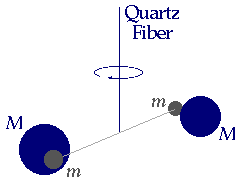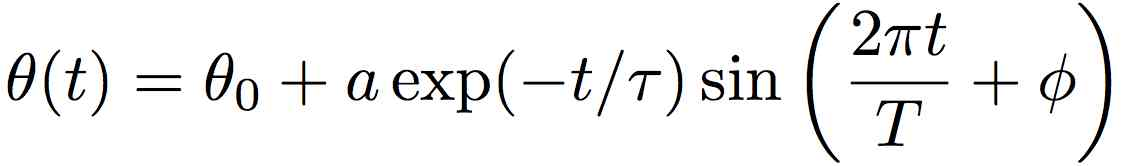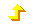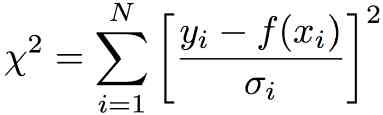Understanding Basic StatisticsFittingExerciseExcelIgorKaleidagraphOriginPower LawsDimensional Analysis

Fitting Exercise

This exercise will help you learn how to use Kaleidagraph or Origin to plot and analyze data.

IntroductionIn 1798 Henry Cavendish conducted an experiment to “weigh the earth.” (Actually, he was interested in the Earth's density, from which he hoped to estimate the time Earth took to cool from a fiery beginning.) He did this by measuring the shift in the equilibrium position of a small dumbbell suspended from a torsion fiber caused by two cannonballs. The balls were placed near the dumbbell so as to make it twist. From the spring constant of the fiber, the geometry and masses of the dumbbell and cannonballs, and the magnitude of the angular shift the cannonballs cause, it is possible to measure the value of G, the universal constant of gravitation. Combined with knowledge of the radius of the earth and the local value of (little)  g, the local acceleration due to gravity, one can deduce the mass of the earth.

Data have been collected for the angular position of the dumbbell as a function of time, and are available by (right) clicking here. Right-click (or control-click) and save the data to a file, for opening in your data analysis program of choice (this preserves the tabs which delimit columns in the file). Alternatively, you may retype them or select them in your browser for pasting into the data analysis program. However, in the latter case be careful that the data show up as numbers in the analysis program, not as text.

The motion is described by a damped sinusoid. Its functional form iswhere the quantities in the equation are defined by

 a (angular) amplitude of oscillation T period of oscillation φ initial phase of the motion τ exponential decay time of the motion θo shift in the equilibrium position of the dumbbell

ExerciseThere are six steps to this exercise. Each task has a help link for Kaleidagraph and Origin, which you may obtain by clicking the link.

1. Load the data ( KG Help, Origin Help ).

2. Plot the data ( KG Help, Origin Help ).

3. Add error bars ( KG Help, Origin Help ) to the plot.

Do the data look like they could represent the motion of a twisting pendulum slowed down by air resistance? Save the plot.

4. Define a fitting function. ( KG Help, Origin Help) that calculates a damped sinusoid. In Kaleidagraph, be careful to check whether you wish the trigonmetric function to be evaluted in radians or degrees. Also be sure to check the Weight Data box in the fit function dialog box.

Fit your data. Be very careful to choose good guesses for your parameters. If you don't, you will very likely get an error like Singular Matrix Error or some such "useful" diagnostic information. In Origin, you can press the Update Plot button to see how you are doing. In Kaleidagraph, there is no such facility.

5. When you have finished the fitting procedure, you should have a graph showing the data with error bars, the fitted curve, and some fit information ( KG Help, Origin Help ). When you perform a proper χ2 fit, the error associated with each fit parameter gives you a sensible estimate of the accuracy of the parameter, as determined by the fitting operation. That's pretty nice. Have a look at your graph and see how well you could eyeball the angular offset. It's tough!

6. Lastly, print out a copy of the graph ( KG Help, Origin Help ). Make the plot area about 4 inches wide by 3 inches high. This is a nice size for taping into a lab notebook.

The Meaning of Fit ParametersThe function χ2 is defined bywhich looks more frightening than it is. This function adds up the differences between each data point and the fitted curve, symbolized here by f. In this equation, the xi are the values of the independent variable. In this example, the independent variable is time. The yi are the values of the dependent variable, which is θ in this problem. The sum is over the N data points.

There are two subtleties. First, the differences are squared, to make them all add up positively. If they weren't, the average would be zero! Second, each difference is divided by the uncertainty of the data point. This means that you get penalized a lot when the curve is far from a "good" data point (that has small uncertainty), and not much at all when the curve is far from a "lousy" data point (that has a large uncertainty).

Since χ2 is the sum of nonnegative quantities, the smallest value it can have is zero. This happens when the curve goes through each and every data point. You might think that this is the best you can do, but you'd be wrong. It means either that you cheat or that you're really sloppy! (Newton cheated with his data and got away with it, because nobody was very sophisticated about this sort of thing when Newton was working out the foundations of mechanics.) Real data have random noise at some level, and this means that they will virtually never lie right on the curve. The uncertainty of a data point is an estimate of the likely discrepancy between the point and its true value. Roughly speaking, a typical data point should be within about one uncertainty ("one error bar") from its true value. This means that each data point should contribute a value of about one to the sum. So the sum should be about N.

It is often more convenient to compute the "per point" value of χ2. This is called the reduced χ2 or χ2 per degree of freedom. It turns out that the best procedure is to divide χ2 not by the number of data points, N, but by N - m, where m is the number of fitting parameters. The number you get should be in the ballpark of unity for a good fit. If it's much smaller than you probably overestimated your errors. If it's much bigger, you may have underestimated your errors or your model may not describe the data very well.

Reduced Chi SquareOrigin reports a value of chisq. Don't be fooled. It is the reduced χ2.

Kaleidagraph reports a value of chi^2. It is χ2. To get the reduced χ2, divide by the number of degrees of freedom (N - m).Copyright © Harvey Mudd College Physics Department 241 Platt Blvd., Claremont, CA 91711 909-621-8024 http://www.physics.hmc.edu/ WebMaster (at) physics.hmc.edu Last modified: 26 August 2012